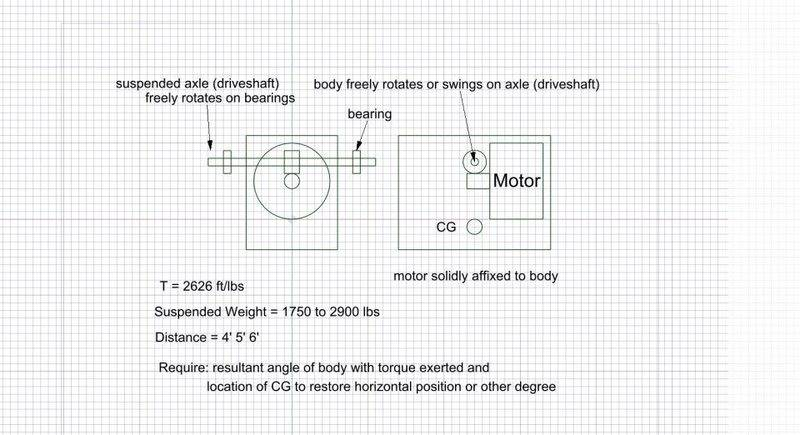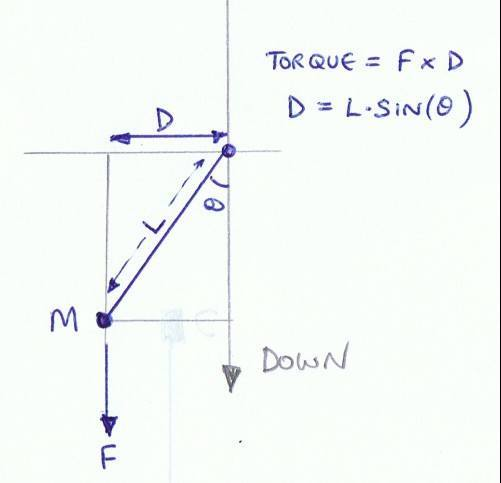# Resultant angle of a suspended body after torque is applied

• Designman
In summary, the body will rotate around the axle at a number of different angles depending on the torque applied.f

#### Designman

A body in an absolute vacuum is suspended from an axle having no friction at the body or at the suspension points.
A power source is affixed to the body which rotates the axle.
At rest the body is horizontal.
The body weighs 4000 lbs.
The center of gravity of the body is vertically 4 ft. below the axle.
The power source applies 4000 ft/lb torque.
How to compute the resultant angle of the body with engine torque applied?
How to compute location of CG to attain a given angle of the body?

A body in an absolute vacuum is suspended from an axle having no friction at the body or at the suspension points.
A power source is affixed to the body which rotates the axle.
At rest the body is horizontal.
The body weighs 4000 lbs.
The center of gravity of the body is vertically 4 ft. below the axle.
The power source applies 4000 ft/lb torque.
How to compute the resultant angle of the body with engine torque applied?
How to compute location of CG to attain a given angle of the body?

Clearly the weight cannot be lifted to θ = 90 as 4000lb * 4ft > 4000 ft lb
so static equilibrium will be reached where 0 < θ < 90

Draw a free body diagram, apply equations of static equilibrium (just ΣM=0 is needed) then form an expression to solve for θ.

Hi billy. The result. At 90 degrees the applied torque equals the weight of the suspended mass. At 45 degrees, 1/2 the suspended weight. It's a simple mathematical relationship! Thanks for your assistance in getting my head around this.

I would have thought the function needed would be a sine, so that at 45° you would get 4000lb x 4ft x sin(45) = 16,000 x 0.707
Alternatively, the weight is 4000lb and if only 4000 ft.lb is available the angle would be such as to make the offset from the axle 1 foot.
4ft x sin(th) = 1ft
th = asin(1/4) = 14.5°

Hi billy. The result. At 90 degrees the applied torque equals the weight of the suspended mass. At 45 degrees, 1/2 the suspended weight. It's a simple mathematical relationship! Thanks for your assistance in getting my head around this.

That's not right. Show your working and we'll see where you went wrong.

Clearly, I am in over my head.
What I require is a formula where I can plug in torque using ft/lbs, horizontal distance of CG from axle in feet or inches, and weight in pounds.
I require degrees from horizontal, and offset of CG to maintain horizontal or another degree.
A lab set up with a pendulum having a low mass rod and ball bearing pivot, and using an accurate pounds gauge, resulted in a 45 degree reading of 50%! That threw me off.
It is extremely important that I get this correct. Your help would be greatly appreciated.

Further, the actual figures I'm working with are T = 2626 ft/lbs Weight = 1750 lbs and 2900 lbs, and D = 4' and 5'.

I think you'll need to provide a diagram I'm not sure I'm imagining your setup right.In the problem as stated nothing would happen at all .

No friction on the axle means no torque reaction which means no forces to move the body .

Please tell us more completely what this problem is all about .The axle is driving a resistance with a resultant torque of 2626 ft/lbs

Lesolee, okay good. The torque is constant at 4' or 1 / 4 x suspended mass. What is the resultant angle after toque is applied?

Sorry, please ignore previous post. The torque applies a constant torque of 1 / 4 at 4' on a suspended vertical mass.
What is the resultant angle after toque is applied?

As far as I can tell, your diagram doesn't match lesolee's.
I agree with Nidium:

In the problem as stated nothing would happen at all .

No friction on the axle means no torque reaction which means no forces to move the body .

Please tell us more completely what this problem is all about .

Also, stalling an electric motor will often destroy it and should be avoided.

The motor is driving an axle which is driving a resistance ( not shown) that generates torque. That torque would spin the body around the axle if the axle was fixed.

Maybe this will help. Think about the anti-torque rotor on a helicopter. But, instead of being a simple torque moment problem, we have a suspended weight.

The motor is driving an axle which is driving a resistance ( not shown) that generates torque. That torque would spin the body around the axle if the axle was fixed.

OK I understand what we are dealing with now .

First observation is that the system is unstable and that there is no single fixed value for the angle of rotation of the block .

Have a think about that while I have my tea .

We have a fixed CG that maintains the body in a horizontal plane when absent of applied torque.

Here is the problem simplified. I think I should have presented the problem like this:
A pendulum bob of mass M, and having a rod of no mass and a length D, pivots from a bearing having no friction.
A force F is applied tangentially to the pendulum's arc to the mass M.
Compute new angle after F is applied to M

Anybody got some thoughts?

In a conversation I asked @Designman to clarify what his actual problem is . This is his reply :

A common driveshaft connects two machines.
Between the machines a chassis hangs freely from that driveshaft.
A power source is rigidly attached to the chassis.
The power source rotates the driveshaft at a constant RPM through a transmission.
The machines offer a constant resistance to rotation.
The center of gravity of the chassis is directly below the driveshaft.
The chassis hangs horizontally.
The weight of the chassis counter reacts the torque applied to the driveshaft.

Friction and startup torque is ignored.
Torque is constant

What is the resultant offset of the chassis with torque applied?

This may seem a trivial problem but in reality it is not .

You have to have a restraint of some kind to stabilise the chassis otherwise it will just swing about in an uncontrolled manner when under load .

A rigid link or a damper system could be used .

Yes, the chassis has a damper.

Any more help would be appreciated. Still stumped.